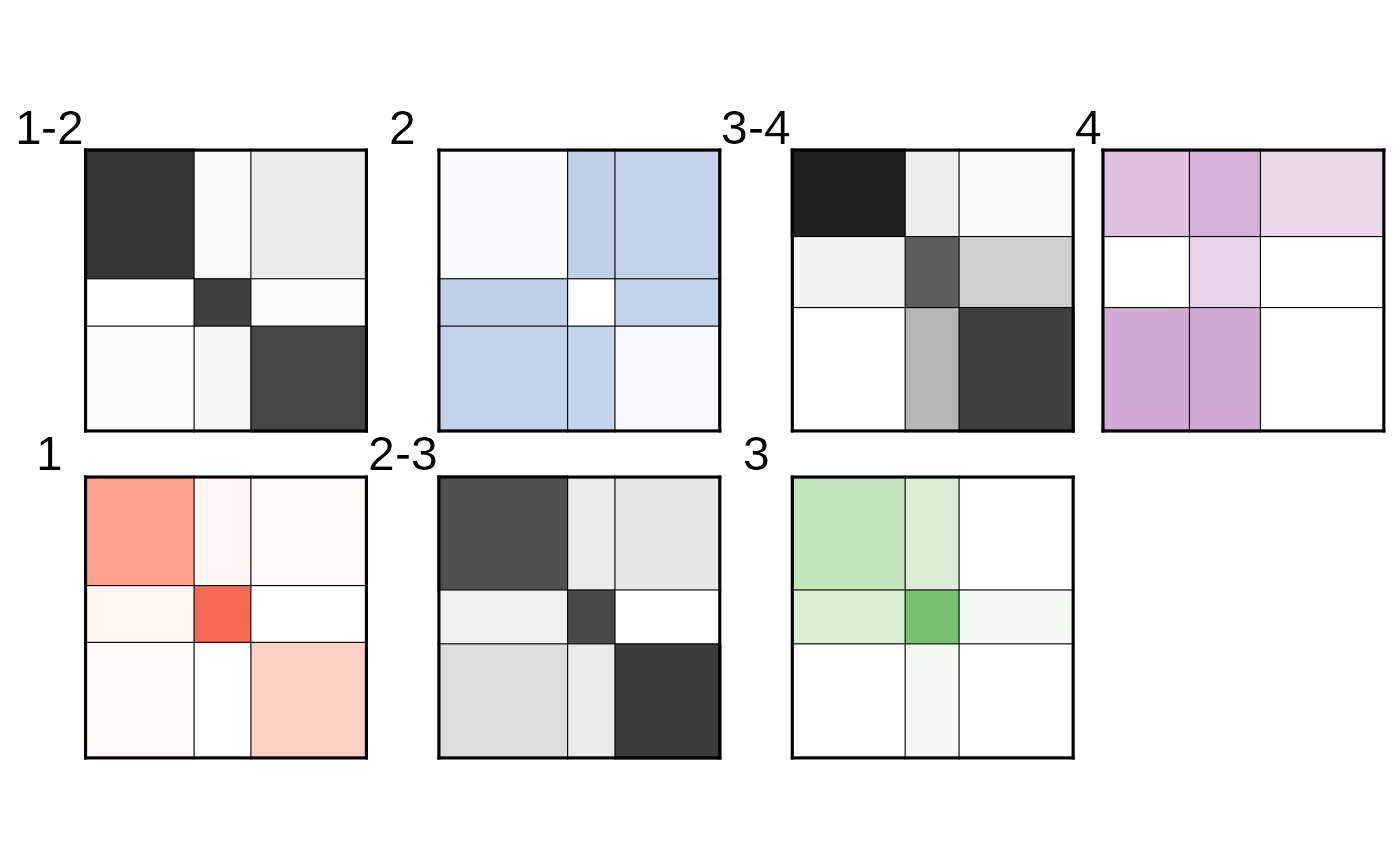library(MLVSBM)

## Presentation of generalized multivel networks

This vignette shows how to use the MLVSBM package to deal with generalized multilevel networks. This is done by modeling these networks with an ad-hoc Stochastic Block Model. The mathematical details can be found at http://www.theses.fr/en/2022UPASM005 (Chapter 2.E).

A diagonal acyclic graph of the model for a graph with $$L$$ levels is provided below.

        A^1             A^{L-1}
|               |
v               v
Z^1 --> Z^2 --> ... --> Z^L
|       |               |
v       v               v
X^1 --> X^2 --> ... --> X^L

A temporal network with the same nodes at each time is a particular case of generalized multilevel networks with diagonal affiliation matrices.

## Description of the data

Assume that we have a generalized multilevel networks with $$4$$ levels, each between $$80$$ and $$100$$ nodes and $$3$$ latent blocks. The description of the levels are the following:

1. An undirected network with an assortative community structure
2. An undirected network with a disassortive structure
3. An undirected network with a core-periphery structure
4. A directed network with an asymmetric structure.

The stability of clusters between levels is quite strong as 80% of the nodes belonging to the same cluster in a level will also be grouped together on the next level.

## Simulating the data

We set the simulation parameters:

n <- 100
L <- 4
alpha <- list(
diag(.4, 3, 3) + .1,
-diag(.3, 3, 3) + .4,
matrix(c(.8, .2, .1,
.4, .4, .1,
.2, .1, .1), 3, 3),
matrix(c(.3, .5, .5,
.1, .4, .5,
.1, .3, .1), 3, 3)
)
alpha[][1,1] <- .8
alpha[][3,3] <- .3
gamma <- lapply(seq(3),
function(m) matrix(c(.8, .1, .1,
.1, .8, .1,
.1, .1, .8), 3, 3, byrow = TRUE))
pi <- list(c(.2, .3, .5), NULL, NULL, NULL)

directed = c(FALSE, FALSE, FALSE, TRUE)

The network can then be simulated.

set.seed(1234)
gmlv <- mlvsbm_simulate_generalized_network(
n = rep(n, 4) - c(20,20,0,0), # Number of nodes
Q = rep(3, 4), # Number of blocks
pi = pi, # Mixture parameters
gamma = gamma, # Affiliation paramters
alpha = alpha, # Connectivity paramters
directed = directed,
distribution = rep("bernoulli", 4))

By doing this we simulated a generalized multilevel network with $$4$$ adjacency matrices and $$3$$ affiliation matrices.

str(gmlv$adjacency_matrix) #> List of 4 #>$ : num [1:80, 1:80] 0 0 0 0 0 0 1 0 1 0 ...
#>  $: num [1:80, 1:80] 0 0 0 1 0 1 1 1 0 0 ... #>$ : num [1:100, 1:100] 0 0 0 1 0 1 0 0 0 1 ...
#>  $: num [1:100, 1:100] 0 1 1 0 0 0 1 1 1 1 ... str(gmlv$affiliation_matrix)
#> List of 3
#>  $: num [1:80, 1:80] 0 0 0 0 0 0 0 0 0 0 ... #>$ : num [1:100, 1:80] 0 0 0 0 0 0 0 0 0 0 ...
A = gmlv$affiliation_matrix, directed = directed, distribution = rep("bernoulli", 4)) then we can fit the model with an initialization of just one block for each level: fit_from_scratch <- mlvsbm_estimate_generalized_network(gmlv, init_clustering = lapply(seq(4), function(x)rep(1, n)), init_method = "merge_split", nb_cores = 1L, fit_options = list(ve = "joint") ) #>  "======= # Blocks : 1, 1, 1, 1, ICL : -12220.6529663515========" #>  "======= # Blocks : 2, 1, 1, 1, ICL : -12127.4888258263========" #>  "======= # Blocks : 2, 2, 1, 1, ICL : -12068.6650821436========" #>  "======= # Blocks : 2, 2, 2, 1, ICL : -11873.9762196481========" #>  "======= # Blocks : 2, 2, 2, 2, ICL : -11534.4113497037========" #>  "======= # Blocks : 3, 2, 2, 2, ICL : -11459.1381283323========" #>  "======= # Blocks : 3, 3, 2, 2, ICL : -11446.4176112849========" #>  "======= # Blocks : 3, 3, 3, 2, ICL : -11403.4416146134========" #>  "======= # Blocks : 3, 3, 3, 3, ICL : -11058.126446938========" #>  "ICL for interdependent levels : -11058.126446938" Or by first fitting an independent SBM on each level, and precising that we need 3 blocks on each level: fit <- mlvsbm_estimate_generalized_network(gmlv, nb_clusters = rep(3, 4), nb_cores = 1L, fit_options = list(ve = "sequential")) #> 0/1: #> 0/1: #> 0/2: #> 0/2: #> 0/3: #> 0/3: #> 0/4: #> 0/4: #>  "====== Searching neighbours...========" #>  "====== Back to desired model size...=======" #>  "======= # Blocks : 3, 3, 3, 3, ICL : -11058.1265367866========" #>  "ICL for interdependent levels : -11058.1265367866" ## Results and analysis We can then plot the fitted structure: plot(fit_from_scratch)Or compared for each level, the inferred clustering with the simulated one with ARI (1 for perfect recovery, 0 for clustering by chance): paste( "ARI for level", seq(L), ":", round(sapply(seq(L), function(l) ARI(x = gmlv$memberships[[l]],
y = fit_from_scratch$Z[[l]])), 2) ) #>  "ARI for level 1 : 1" "ARI for level 2 : 1" "ARI for level 3 : 0.96" #>  "ARI for level 4 : 1" Or compare the inferred clustering from our two methods of inference with ARI: paste( "ARI for level", seq(L), ":", round(sapply(seq(L), function(l) ARI(x = fit$Z[[l]],
y = fit_from_scratch\$Z[[l]])), 2)
)
#>  "ARI for level 1 : 1" "ARI for level 2 : 1" "ARI for level 3 : 1"
#>  "ARI for level 4 : 1"